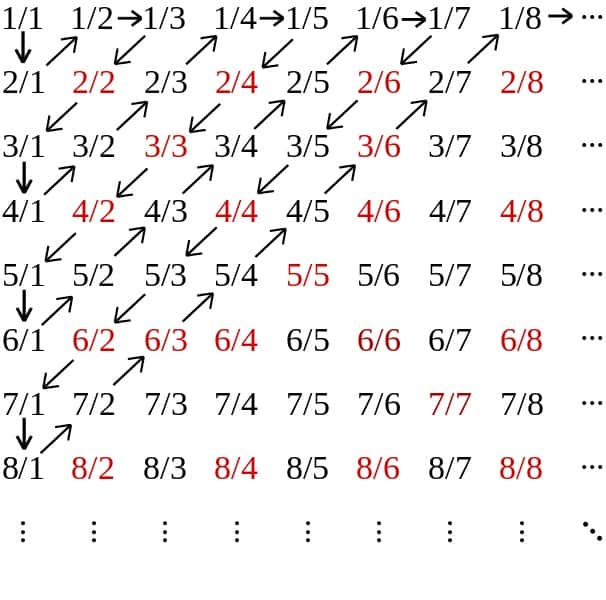If you’ve ever asked what the largest number is during a maths lesson it is quite likely that some bright spark piped up with an answer along the lines of: “That’s easy! It’s infinity of course!”

The only problem with infinity is that it isn’t a number as such, as demonstrated by the conversation below between two bright sparks.

Bright spark one: “Infinity is the biggest number in the world, that’s easy!”

Bright spark two: “Well I’ve got a bigger number for you – infinity plus one!”

Bright spark one again: “I’ve got a number to beat yours – infinity plus one, times a million!”

Conversation goes on like this for what seems like an infinite amount of time until neither bright spark has arrived at the world’s biggest number.

Before long the two bright sparks have realised that infinity is not really a number at all, it is more of a concept. What no one has told the two bright sparks yet is about the shocking idea that there are different sizes of infinity! So how do we calculate the largest number?

### The infinity of the counting numbers

The simplest way of creating a set of numbers which is infinite in size is by counting up in whole numbers. This set of numbers is called the natural numbers and obviously it is infinite in size as we can carry on counting forever. The symbolis used to label this set and it stands for ‘natural numbers’.Let us now look at a different list of numbers and call this set(our own label):The setis also infinite in size, but appears to contain one less number than. Are they the same size?

We can show thatandare in fact the same size by showing that there is a one to one correspondence between the elements ofand the elements of.Up till now we would have said that the size ofwas simply infinity, which is written like a number eight on its side:.

However we are about to find out that there are different sizes of infinity, and so we now label the size ofas beingwhich is pronounced as ‘aleph zero’.is the smallest size of infinity, and our setalso has size.

### Other sets which have the sizeThere are many other sets of numbers which have the infinite size of. These include the set of positive even whole numbers, and also what is known as the set of rational numbers. Rational numbers are all the numbers which can be written as fractions. If a set of numbers has the sizeit is said to be countable.

We can write down every possible fraction into a table like the one below. Equivalent fractions might appear more than once, for examplehowever we can easily remove any repeats from the table. We can then draw on a diagonal pattern which will allow us to put our fractions into a list. We are now left with a neat list of fractionsIf we have a list of fractions they can be counted and the rational numbers are therefore said to be countable.By Cronholm144 (Own work) [GFDL (http://www.gnu.org/copyleft/fdl.html), CC-BY-SA-3.0 (http://creativecommons.org/licenses/by-sa/3.0/) via Wikimedia Commons

How do we find a size of infinity which is bigger than?

Not every number can be written as a fraction. Numbers that can’t be written as fractions are called irrational numbers. Well known examples includeand surds such asand.

The decimal expansions of irrational numbers like(3.1415926535…) go on forever, and these numbers can never be written as fractions, even though people like to useas an approximation for.

Let’s now look at the set of all numbers which are between 0 and 1. This set will include rational numbers such asas well as irrational numbers such asThis set of numbers is clearly infinite in size, as we can always think of more and more numbers which are contained in the interval (0,1).

In 1873 a German mathematician called Georg Cantor invented a very clever proof that the set of all real numbers in the interval (0,1) has a size which is a bigger infinity than the size of the set of the natural numbers.

### Summary of Cantor’s famous diagonal argument.

Let’s assume that the size of the set of all real numbers in the interval (0,1) is the same size as. We could then make a list attempting to count up through the real numbers between 0 and 1. It could look something like this if we weren’t being very logical:Cantor’s really clever next step was to construct a new number which isn’t on the list. Cantor’s argument will work either if we use a list like the one above, or even if we painstakingly tried to make a logical list which attempted to capture every number between 0 and 1:

### Cantor’s clever way of choosing a number not on the list.

This new number is clearly not on the list and Cantor had found a contradiction – Cantor showed that you can never make a one to one correspondence between the natural numbers and the real numbers in the interval (0,1). Cantor had proved that the size of the real numbers is bigger than the size of the natural numbers! The real numbers are uncountable! There are different sizes of infinity!

In conclusion, the answer to the question of what is the largest number in the world is not straightforward. In a nutshell, there is no largest number, you can keep counting forever. But you can also find two groups of numbers – both infinite in size, but also different in size to each other. It is really incredible to think about!